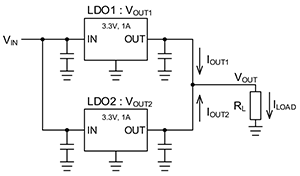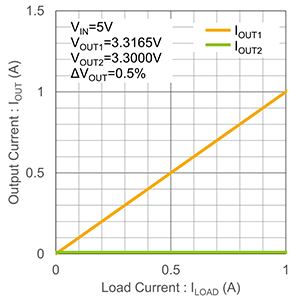# About Parallel Connections of LDO Linear Regulators

2020.10.21

・By connecting LDOs in parallel, it is possible to increase the output current and avoid exceeding the allowable dissipation limit.

・Merely connecting the outputs of LDOs directly does not result in correct load sharing, due to differences in output voltages.

LDO linear regulators are linear-type step-down voltage regulators with low saturation (Low Dropout: LDO), often simply referred to as LDOs, and are currently the mainstream of linear regulators. They have a simple design and offer numerous advantages with respect to number of components, size, and cost, and so even with the emergence of switching regulators in recent years, they are power supply ICs that are widely used in various applications.

The allowable dissipation of LDO linear regulators (hereafter “LDOs”) in general is at most a few watts. For example, an LDO with a 5 V input and 3.3 V output has an output current of about 1 A; for outputs exceeding this, in recent years there has been a trend of opting for a switching regulator. However, there are methods in which LDOs can continue to be used while increasing the output current and avoiding losses exceeding the allowable dissipation. One such method involves parallel connection of LDOs.

## Parallel Connection of LDO Linear Regulators

As a technique, LDO parallel connections are quite old. Basically and ideally, when for example two 1 A LDOs are put in parallel, twice the current, or 2 A, is obtained, and from the standpoint of distribution of losses, with two devices in parallel, the loss in each is half as great.

However, if the outputs of LDOs are simply connected directly, the expected addition of output currents and halving of losses will not occur. Below are shown an example of a circuit in which outputs are directly connected in parallel, together with a graph example indicating the output currents for the load currents of each LDO. Here two LDOs capable of a VIN of 5 V, a VOUT of 3.3 V, and an IOUT of 1 A are connected in parallel. In principle, for a given load current ILOAD, the IOUT of each of the LDOs should be 1/2ILOAD, but as the graph indicates, in this example nearly all of the load current is supplied by the LDO1, and almost none comes from the LDO2. As a result, in this circuit the LDO1 reaches its IOUT supply capacity of 1A, so that even with two devices connected in parallel, a greater IOUT cannot be supplied.This is caused by the difference in the output voltages of the LDOs connected in parallel. Even when LDOs have the same nominal output voltage value, in actuality there is variation in the output voltage within a guaranteed range of values, In this example, VOUT1, which is the output of LDO1, is 3.3165 V, and VOUT2 for LDO2 is 3.3000 V, for a difference of 0.0165 V = 0.5%.

When lines with different voltage values are connected, current will flow from the line with the higher voltage value. Similarly, when the outputs of LDOs with different output values are directly connected, current will be supplied from the LDO with the higher output voltage. In this example, LDO1 supplies the output current, just as shown in the graph. In general, allowed differences in the output voltages of LDOs are stipulated to be within plus/minus a few percent of the nominal value, and as a practical matter, it cannot be expected that LDOs connected in parallel will have the same output voltages. Hence in order to connect LDOs in parallel, a circuit must be devised that satisfactorily divides the output current among the parallel LDOs.

Methods of LDO parallel connection include a method that uses diodes and a method that uses resistors. Each of these methods will be explained subsequently.